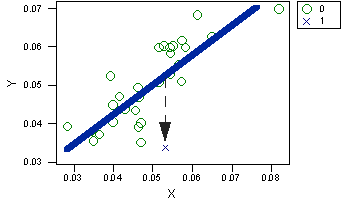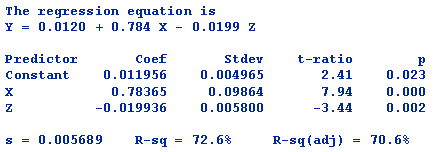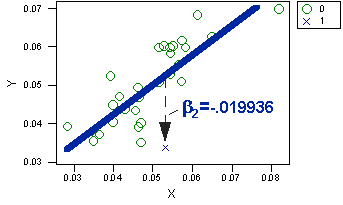Regression Point Displacement

# Regression Point Displacement Analysis

## Statistical Requirements

The notation for the Regression Point Displacement (RPD) design shows that the statistical analysis requires:

• a posttest score
• a pretest score
• a variable to represent the treatment group (where 0=comparison and 1=program)These requirements are identical to the requirements for the Analysis of Covariance model. The only difference is that the RPD design only has a single treated group score.The figure shows a bivariate (pre-post) distribution for a hypothetical RPD design of a community-based AIDS education program. The new AIDS education program is piloted in one particular county in a state, with the remaining counties acting as controls. The state routinely publishes annual HIV positive rates by county for the entire state. The x-values show the HIV-positive rates per 1000 people for the year preceding the program while the y-values show the rates for the year following it. Our goal is to estimate the size of the vertical displacement of the treated unit from the regression line of all of the control units, indicated on the graph by the dashed arrow. The model we’ll use is the Analysis of Covariance (ANCOVA) model stated in regression model form:

$$y_{i}=\beta_{0}+\beta_{1} X_{i}+\beta_{2} Z_{i}+e_{i}$$

where:

• yi = outcome score for the ith unit

• β0 = coefficient for the intercept

• β1 = pretest coefficient

• β2 = mean difference for treatment

• xi = covariate

• Zi = dummy variable for treatment (0 = control, 1 = treatment [n=1])

• ei = residual for the ith unit

When we fit the model to our simulated data, we obtain the regression table shown below:The coefficient associated with the dichotomous treatment variable is the estimate of the vertical displacement from the line. In this example, the results show that the program lowers HIV positive rates by .019 and that this amount is statistically significant. This displacement is shown in the results graph:For more details on the statistical analysis of the RPD design, you can view an entire paper on the subject entitled, “The Regression Point Displacement Design for Evaluating Community-Based Pilot Programs and Demonstration Projects”.